# Using model builder for summary statistics and setting ranges to group by

1017
4
03-23-2019 04:50 AMNew Contributor II

Hi

I am trying to perform a summary on a poly line dataset. This data contains an attribute for the year the section was laid and the diameter of the section. I want to sum the length of these sections group them by both year and band. However I want to group the bands and years e.g  year laid 2000-2010 and diameter 150-300 mm. I have created a model which does what I want but I was wondering if there was an easier way to carry out this task. I was also wondering if there was a way to modify the model so that the user could can the year bands and diameters in a table of spreadsheet. I am new to the model builder. Maybe there is a better way to do what I am trying to do. See attached images.

Thanks

Ciaran

Tags (4)
1 Solution

Accepted SolutionsbyMVP Esteemed Contributor

You can make an model input variable a multi value list, one variable for each of your classify variables.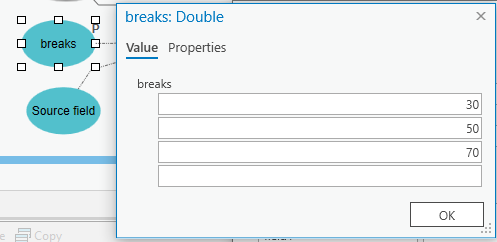Then you can use that multi value list in Calculate Field, inside the code block splitting it into a Python list with “;”.split().

Here is a Calculate Field expression I came up with that seems to do what you want. Dan Patterson‌ numpy is pretty handy!

``````# Calculate Field expression, specifying to update field "class"
# Source field is the name of the field we want to classify
classify(!%Source field%!)

# Code block
# breaks is a multivalue model variable I created of type Double
import numpy as np
breaks = sorted([float(k) for k in "%breaks%".split(";")])
breaklab = ["<{}".format(breaks)]
breaklab += ["{} up to {}".format(breaks[k], breaks[k+1]) for k in range(len(breaks)-1)]
breaklab += [">={}".format(breaks[-1])]
breaks = np.array(breaks)
def classify(vv):
p = np.searchsorted(breaks, vv, 'right')
return breaklab[p]‍‍‍‍‍‍‍‍‍‍‍‍‍‍‍‍‍‍‍‍‍‍‍‍‍‍‍‍‍‍‍‍‍‍‍‍‍‍‍‍‍‍‍‍‍‍‍‍‍‍‍‍‍‍‍‍‍‍‍‍‍‍‍‍‍‍‍‍‍‍‍‍‍‍‍‍‍‍‍‍‍‍‍‍‍‍‍‍‍‍‍‍‍‍‍‍‍‍‍‍‍‍‍‍``````4 RepliesbyMVP Esteemed Contributor

You can make an model input variable a multi value list, one variable for each of your classify variables.Then you can use that multi value list in Calculate Field, inside the code block splitting it into a Python list with “;”.split().

Here is a Calculate Field expression I came up with that seems to do what you want. Dan Patterson‌ numpy is pretty handy!

``````# Calculate Field expression, specifying to update field "class"
# Source field is the name of the field we want to classify
classify(!%Source field%!)

# Code block
# breaks is a multivalue model variable I created of type Double
import numpy as np
breaks = sorted([float(k) for k in "%breaks%".split(";")])
breaklab = ["<{}".format(breaks)]
breaklab += ["{} up to {}".format(breaks[k], breaks[k+1]) for k in range(len(breaks)-1)]
breaklab += [">={}".format(breaks[-1])]
breaks = np.array(breaks)
def classify(vv):
p = np.searchsorted(breaks, vv, 'right')
return breaklab[p]‍‍‍‍‍‍‍‍‍‍‍‍‍‍‍‍‍‍‍‍‍‍‍‍‍‍‍‍‍‍‍‍‍‍‍‍‍‍‍‍‍‍‍‍‍‍‍‍‍‍‍‍‍‍‍‍‍‍‍‍‍‍‍‍‍‍‍‍‍‍‍‍‍‍‍‍‍‍‍‍‍‍‍‍‍‍‍‍‍‍‍‍‍‍‍‍‍‍‍‍‍‍‍‍``````New Contributor II

Curtis,

Thanks for the information but i must be doing something stupid. I have tried to simplify this to test. So i created a new feature class with the same structure as your example and with the same names. When i try to run the model i get an error about value type. I have set the to type double. See attached images which will hopefulyl help.

Regards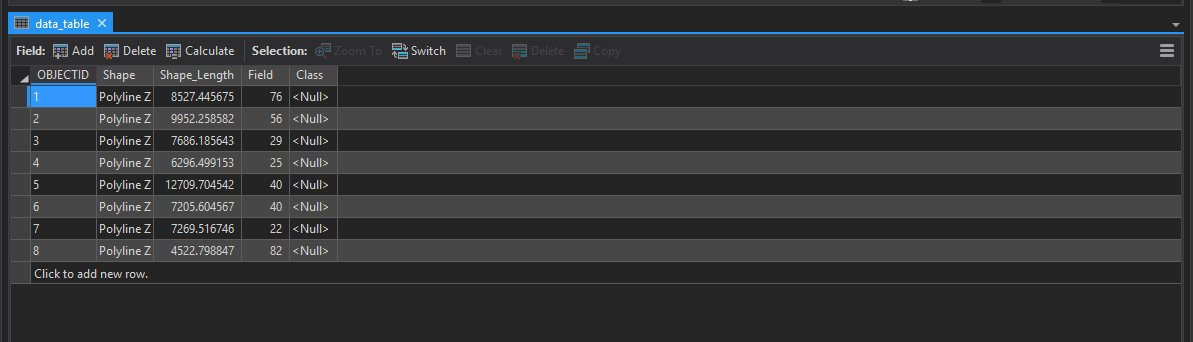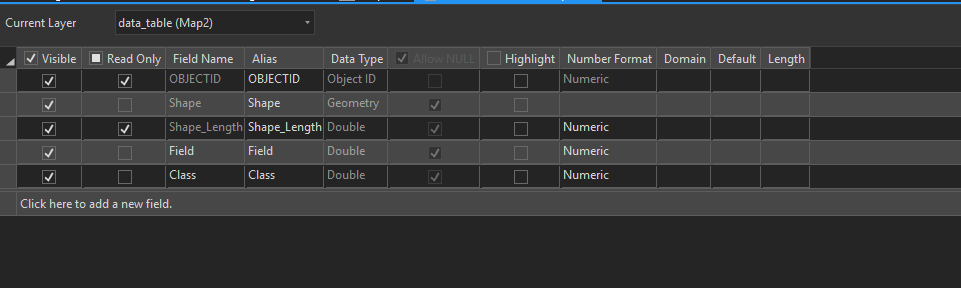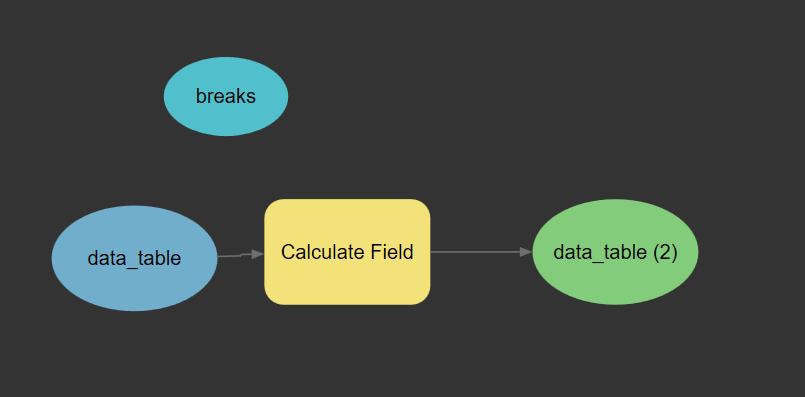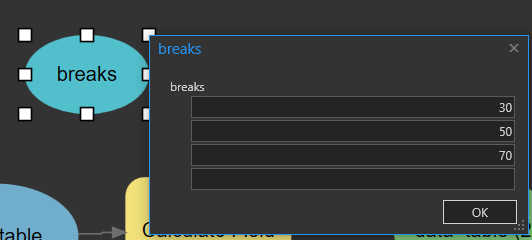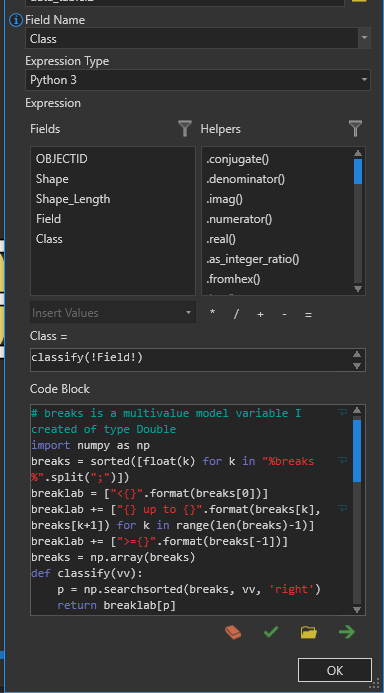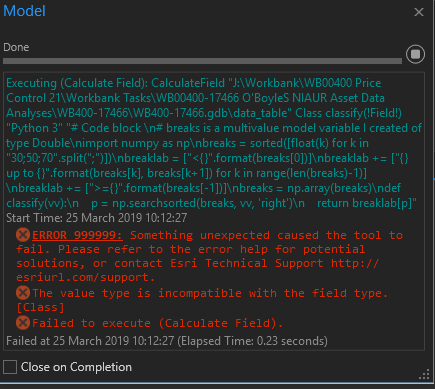CiaranNew Contributor II

I have got this working now thanks.

I didn't realise the coulmn that is being updated has to be of datatype text/string.

Thanks againNew Contributor III

i know this is an old post but i am doing something similar, but instead of multivalue variable i am using Table of Values variable. and i need to split the variables so that i could use in them for field calculation.

I have posted a question here in communities would love to pick your brains on the topic

Thanks

Hussain